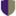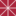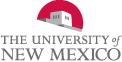# Algebra Commons™

1,291 Full-Text Articles 1,340 Authors 454,981 Downloads131 Institutions

## All Articles in Algebra

1,291 full-text articles. Page 48 of 49.

Recognizing Graph Theoretic Properties With Polynomial Ideals, 2010University of California - Davis

#### Recognizing Graph Theoretic Properties With Polynomial Ideals, Jesus A. De Loera, Christopher J. Hillar, Peter N. Malkin, Mohamed Omar

##### All HMC Faculty Publications and Research

Many hard combinatorial problems can be modeled by a system of polynomial equations. N. Alon coined the term polynomial method to describe the use of nonlinear polynomials when solving combinatorial problems. We continue the exploration of the polynomial method and show how the algorithmic theory of polynomial ideals can be used to detect k-colorability, unique Hamiltonicity, and automorphism rigidity of graphs. Our techniques are diverse and involve Nullstellensatz certificates, linear algebra over finite fields, Gröbner bases, toric algebra, convex programming, and real algebraic geometry.

Linear Stochastic State Space Theory In The White Noise Space Setting, 2010Chapman University

#### Linear Stochastic State Space Theory In The White Noise Space Setting, Daniel Alpay, David Levanony, Ariel Pinhas

##### Mathematics, Physics, and Computer Science Faculty Articles and Research

We study state space equations within the white noise space setting. A commutative ring of power series in a countable number of variables plays an important role. Transfer functions are rational functions with coefficients in this commutative ring, and are characterized in a number of ways. A major feature in our approach is the observation that key characteristics of a linear, time invariant, stochastic system are determined by the corresponding characteristics associated with the deterministic part of the system, namely its average behavior.

Linear Stochastic Systems: A White Noise Approach, 2010Chapman University

#### Linear Stochastic Systems: A White Noise Approach, Daniel Alpay, David Levanony

##### Mathematics, Physics, and Computer Science Faculty Articles and Research

Using the white noise setting, in particular the Wick product, the Hermite transform, and the Kondratiev space, we present a new approach to study linear stochastic systems, where randomness is also included in the transfer function. We prove BIBO type stability theorems for these systems, both in the discrete and continuous time cases. We also consider the case of dissipative systems for both discrete and continuous time systems. We further study ℓ1-ℓ2 stability in the discrete time case, and L2-L∞ stability in the continuous time case.

Discrete-Time Multi-Scale Systems, 2010Chapman University

#### Discrete-Time Multi-Scale Systems, Daniel Alpay, Mamadou Mboup

##### Mathematics, Physics, and Computer Science Faculty Articles and Research

We introduce multi-scale filtering by the way of certain double convolution systems. We prove stability theorems for these systems and make connections with function theory in the poly-disc. Finally, we compare the framework developed here with the white noise space framework, within which a similar class of double convolution systems has been defined earlier.

Review: Quantization Of Hamiltonian-Type Lie Algebras, 2010Pomona College

#### Review: Quantization Of Hamiltonian-Type Lie Algebras, Gizem Karaali

##### Pomona Faculty Publications and Research

No abstract provided.

2010Pomona College

#### Review: Classification Of Quasi-Trigonometric Solutions Of The Classical Yang-Baxter Equation, Gizem Karaali

##### Pomona Faculty Publications and Research

No abstract provided.

2010Pomona College

#### Review: The Semi-Dynamical Reflection Equation: Solutions And Structure Matrices, Gizem Karaali

##### Pomona Faculty Publications and Research

No abstract provided.

2010Pomona College

#### Review: Intertwining Symmetry Algebras Of Quantum Superintegrable Systems, Gizem Karaali

##### Pomona Faculty Publications and Research

No abstract provided.

Some New Classes Of Complex Symmetric Operators, 2010Pomona College

#### Some New Classes Of Complex Symmetric Operators, Stephan Ramon Garcia, Warren R. Wogen

##### Pomona Faculty Publications and Research

We say that an operator $T \in B(H)$ is complex symmetric if there exists a conjugate-linear, isometric involution $C:H\to H$ so that $T = CT^*C$. We prove that binormal operators, operators that are algebraic of degree two (including all idempotents), and large classes of rank-one perturbations of normal operators are complex symmetric. From an abstract viewpoint, these results explain why the compressed shift and Volterra integration operator are complex symmetric. Finally, we attempt to describe all complex symmetric partial isometries, obtaining the sharpest possible statement given only the data $(\dim \ker T, \dim \ker T^*)$.

Krein Systems And Canonical Systems On A Finite Interval: Accelerants With A Jump Discontinuity At The Origin And Continuous Potentials, 2010Chapman University

#### Krein Systems And Canonical Systems On A Finite Interval: Accelerants With A Jump Discontinuity At The Origin And Continuous Potentials, Daniel Alpay, I. Gohberg, M. A. Kaashoek, L. Lerer, A. Sakhnovich

##### Mathematics, Physics, and Computer Science Faculty Articles and Research

This paper is devoted to connections between accelerants and potentials of Krein systems and of canonical systems of Dirac type, both on a finite interval. It is shown that a continuous potential is always generated by an accelerant, provided the latter is continuous with a possible jump discontinuity at the origin. Moreover, the generating accelerant is uniquely determined by the potential. The results are illustrated on pseudo-exponential potentials. The paper is a continuation of the earlier paper of the authors  dealing with the direct problem for Krein systems.

Regular Functions On The Space Of Cayley Numbers, 2010University of Florence

#### Regular Functions On The Space Of Cayley Numbers, Graziano Gentili, Daniele C. Struppa

##### Mathematics, Physics, and Computer Science Faculty Articles and Research

In this paper we present a new definition of regularity on the space Ç of Cayley numbers (often referred to as octonions), based on a Gateaux-like notion of derivative. We study the main properties of regular functions, and we develop the basic elements of a function theory on Ç. Particular attention is given to the structure of the zero sets of such functions.

New Classes Of Neutrosophic Linear Algebras, 2010University of New Mexico

#### New Classes Of Neutrosophic Linear Algebras, Florentin Smarandache, W.B. Vasantha Kandasamy, K. Ilanthenral

##### Mathematics and Statistics Faculty and Staff Publications

In this book we introduce mainly three new classes of linear algebras; neutrosophic group linear algebras, neutrosophic semigroup linear algebras and neutrosophic set linear algebras. The authors also define the fuzzy analogue of these three structures. This book is organized into seven chapters. Chapter one is introductory in content. The notion of neutrosophic set linear algebras and neutrosophic neutrosophic set linear algebras are introduced and their properties analysed in chapter two. Chapter three introduces the notion of neutrosophic semigroup linear algebras and neutrosophic group linear algebras. A study of their substructures are systematically carried out in this chapter. The fuzzy ...

Interval Linear Algebra, 2010University of New Mexico

#### Interval Linear Algebra, Florentin Smarandache, W.B. Vasantha Kandasamy

##### Mathematics and Statistics Faculty and Staff Publications

This Interval arithmetic or interval mathematics developed in 1950’s and 1960’s by mathematicians as an approach to putting bounds on rounding errors and measurement error in mathematical computations. However no proper interval algebraic structures have been defined or studies. In this book we for the first time introduce several types of interval linear algebras and study them. This structure has become indispensable for these concepts will find applications in numerical optimization and validation of structural designs. In this book we use only special types of intervals and introduce the notion of different types of interval linear algebras and ...

Rank Distance Bicodes And Their Generalization, 2010University of New Mexico

#### Rank Distance Bicodes And Their Generalization, Florentin Smarandache, W.B. Vasantha Kandasamy, N. Suresh Babu, R.S. Selvaraj

##### Mathematics and Statistics Faculty and Staff Publications

In this book the authors introduce the new notion of rank distance bicodes and generalize this concept to Rank Distance n-codes (RD n-codes), n, greater than or equal to three. This definition leads to several classes of new RD bicodes like semi circulant rank bicodes of type I and II, semicyclic circulant rank bicode, circulant rank bicodes, bidivisible bicode and so on. It is important to mention that these new classes of codes will not only multitask simultaneously but also they will be best suited to the present computerised era. Apart from this, these codes are best suited in cryptography ...

Interval Groupoids, 2010University of New Mexico

#### Interval Groupoids, Florentin Smarandache, W.B. Vasantha Kandasamy, Moon Kumar Chetry

##### Mathematics and Statistics Faculty and Staff Publications

This book introduces several new classes of groupoid, like polynomial groupoids, matrix groupoids, interval groupoids, polynomial interval groupoids, matrix interval groupoids and their neutrosophic analogues.

Proposed Problems Of Mathematics (Vol. Ii), 2010University of New Mexico

#### Proposed Problems Of Mathematics (Vol. Ii), Florentin Smarandache

##### Mathematics and Statistics Faculty and Staff Publications

The first book of “Problèmes avec et sans … problèmes!” was published in Morocco in 1983. I collected these problems that I published in various Romanian or foreign magazines (amongst which: “Gazeta Matematică”, magazine which formed me as problem solver, “American Mathematical Monthly”, “Crux Mathematicorum” (Canada), “Elemente der Mathematik” (Switzerland), “Gaceta Matematica” (Spain), “Nieuw voor Archief” (Holland), etc. while others are new proposed problems in this second volume.

These have been created in various periods: when I was working as mathematics professor in Romania (1984-1988), or co-operant professor in Morocco (1982-1984), or emigrant in the USA (1990-1997). I thank to the ...

Neutrosophic Bilinear Algebras And Their Generalizations, 2010University of New Mexico

#### Neutrosophic Bilinear Algebras And Their Generalizations, Florentin Smarandache, W.B. Vasantha Kandasamy

##### Mathematics and Statistics Faculty and Staff Publications

This book introduces the concept of neutrosophic bilinear algebras and their generalizations to n-linear algebras, n>2. This book has five chapters. The reader should be well-versed with the notions of linear algebras as well as the concepts of bilinear algebras and n- linear algebras. Further the reader is expected to know about neutrosophic algebraic structures as we have not given any detailed literature about it. The first chapter is introductory in nature and gives a few essential definitions and references for the reader to make use of the literature in case the reader is not thorough with the basics ...

Families Of Symmetries As Efficient Models Of Resource Binding, 2010Institute for Logic, Language and Computation - Amsterdam

#### Families Of Symmetries As Efficient Models Of Resource Binding, Vincenzo Ciancia, Alexander Kurz, Ugo Montanari

##### Engineering Faculty Articles and Research

Calculi that feature resource-allocating constructs (e.g. the pi-calculus or the fusion calculus) require special kinds of models. The best-known ones are presheaves and nominal sets. But named sets have the advantage of being finite in a wide range of cases where the other two are infinite. The three models are equivalent. Finiteness of named sets is strictly related to the notion of finite support in nominal sets and the corresponding presheaves. We show that named sets are generalisd by the categorical model of families, that is, free coproduct completions, indexed by symmetries, and explain how locality of interfaces gives ...

On Universal Algebra Over Nominal Sets, 2010Chapman University

#### On Universal Algebra Over Nominal Sets, Alexander Kurz, Daniela Petrişan

##### Engineering Faculty Articles and Research

We investigate universal algebra over the category Nom of nominal sets. Using the fact that Nom is a full re ective subcategory of a monadic category, we obtain an HSP-like theorem for algebras over nominal sets. We isolate a uniform' fragment of our equational logic, which corresponds to the nominal logics present in the literature. We give semantically invariant translations of theories for nominal algebra and NEL into uniform' theories and systematically prove HSP theorems for models of these theories.

On Coalgebras Over Algebras, 2010University Politehnica of Bucharest

#### On Coalgebras Over Algebras, Adriana Balan, Alexander Kurz

##### Engineering Faculty Articles and Research

We extend Barr’s well-known characterization of the final coalgebra of a Set-endofunctor as the completion of its initial algebra to the Eilenberg-Moore category of algebras for a Set-monad M for functors arising as liftings. As an application we introduce the notion of commuting pair of endofunctors with respect to the monad M and show that under reasonable assumptions, the final coalgebra of one of the endofunctors involved can be obtained as the free algebra generated by the initial algebra of the other endofunctor.